# Wiener-Hopf method

(Redirected from Wiener–Hopf method)
Jump to: navigation, search

A method for solving functional equations of the type: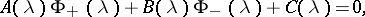(1)

whereare given functions of a complex variable, analytic in a strip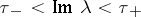, andand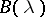are non-zero in this strip. The functions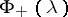and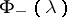are unknown functions of the complex variablewhich tend to zero as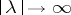and are to be determined,being analytic for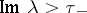andbeing analytic for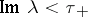. Equation (1) must be satisfied in the entire strip of analyticity.

The Wiener–Hopf method is based on the following two theorems.

1) A function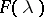which is analytic in the stripand uniformly tends to zero ascan be represented inside this strip as a sum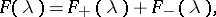where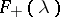is analytic in the half-plane, while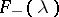is analytic in the half-plane.

2) A functionwhich is analytic and non-zero in the stripand which uniformly tends to one in this strip asis representable in the given strip as a product: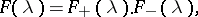(2)

whereandare analytic and non-zero in the half-planesand, respectively. The representation (2) is often called a factorization of the function.

The fundamental idea of the Wiener–Hopf method is that it is possible to factorize the function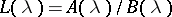; in other words, the method is based on the assumption that a representation(3)

is possible. Using (3), equation (1) may be written as: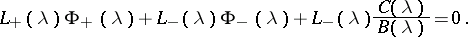Sinceis analytic inside the strip, one has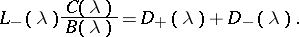(4)

Using (4), one finally obtains equation (1) in the form(5)

The left-hand side of (5) represents a function which is analytic for, while the right-hand side is a function which is analytic for. Since they have a common strip of analyticity in which condition (5) is satisfied, there exists a unique entire function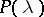which is identical with the left-hand and right-hand sides of (5), respectively, in their domains of analyticity. Hence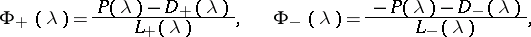i.e. the solution of (1) is unique up to an entire function. If the order of growth ofandis bounded at infinity,must be a polynomial. The functions sought are then determined uniquely up to constants, which are calculated by imposing additional conditions.

The Wiener–Hopf method was developed in  to solve special type integral equations (cf. Wiener–Hopf equation). It subsequently found extensive use in various problems of mathematical physics .